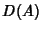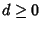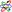## Hausdorff Dimension

Letbe a Subset of a Metric Space. Then the Hausdorff dimensionofis the Infimum ofsuch that the-dimensional Hausdorff Measure ofis 0. Note that this need not be an Integer.

In many cases, the Hausdorff dimension correctly describes the correction term for a resonator with Fractal Perimeter in Lorentz's conjecture.However, in general, the proper dimension to use turns out to be the Minkowski-Bouligand Dimension (Schroeder 1991).

References

Federer, H. Geometric Measure Theory. New York: Springer-Verlag, 1969.

Hausdorff, F. ``Dimension und äußeres Maß.'' Math. Ann. 79, 157-179, 1919.

Ott, E. ``Appendix: Hausdorff Dimension.'' Chaos in Dynamical Systems. New York: Cambridge University Press, pp. 100-103, 1993.

Schroeder, M. Fractals, Chaos, Power Laws: Minutes from an Infinite Paradise. New York: W. H. Freeman, pp. 41-45, 1991.